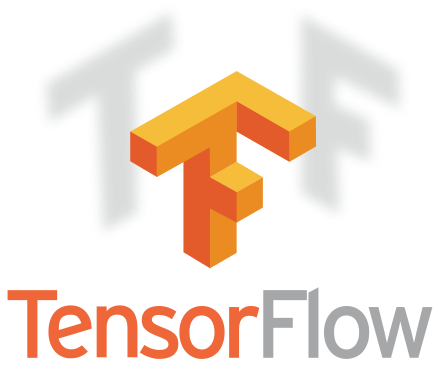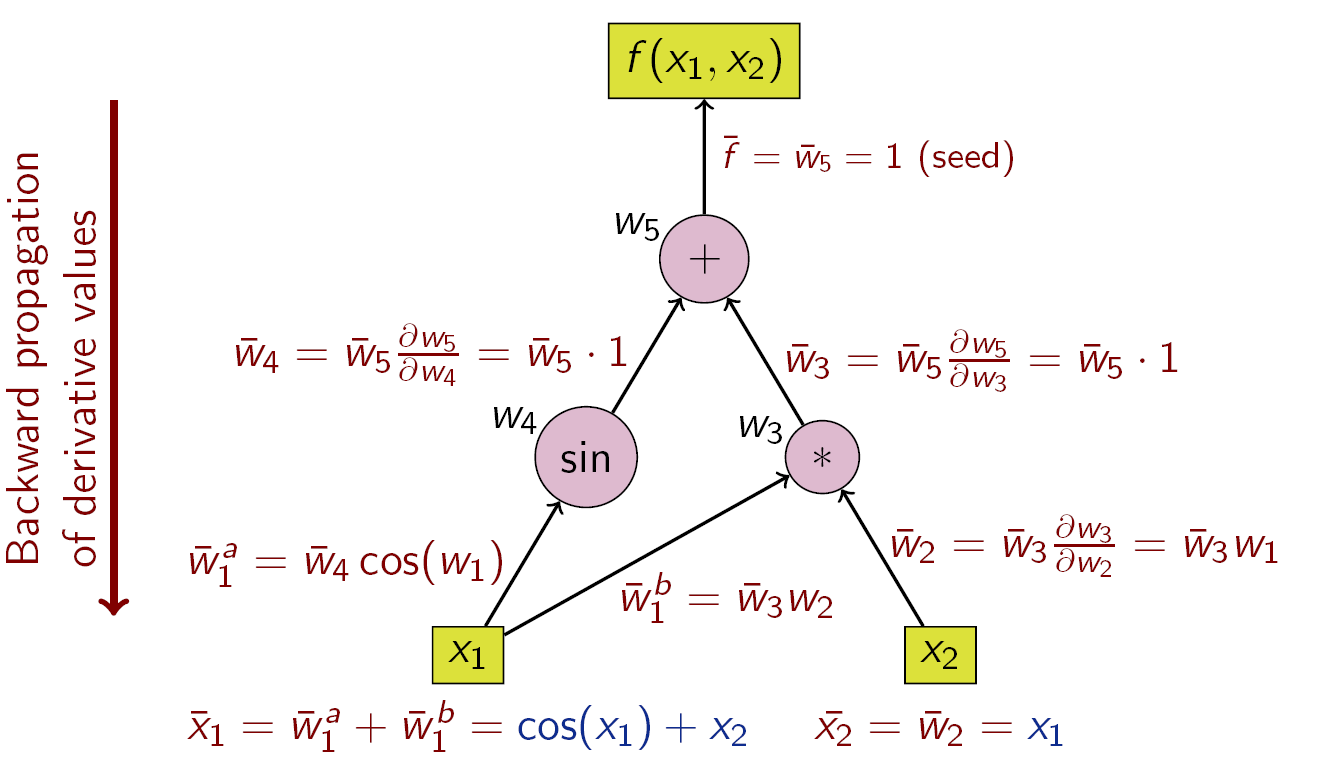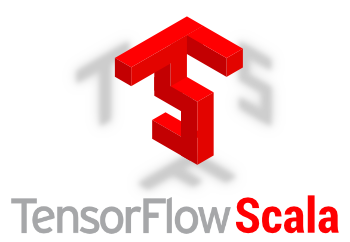# Introduction

Summary

Since v1.5.2, DynaML has moved towards closer integration with Google Tensorflow. This is done via the Tensorflow for Scala project. The DynaML tensorflow package builds on Tensorflow for Scala and provides a high level API containing several convenience routines and building blocks for deep learning.Tensorflow is a versatile and general computational framework for working with tensors and computational graphs. It provides tensor primitives as well as the ability to define transformations on them. Under the hood, these transformations are baked into a computational graph. Obtaining results of computations now becomes a job of evaluating the relevant nodes of these graphs.courtesy Wikipedia.

It turns out that representing computation in this manner is advantageous when you need to compute derivatives of arbitrary functions with respect to any inputs. Tensorflow has the ability to evaluate/execute computational graphs on any hardware architecture, freeing the user from worrying about those details.

The tensorflow API is roughly divided into two levels.

• Low level: Tensor primitives, variables, placeholders, constants, computational graphs.
• High level: Models, estimators etc.

## Tensorflow for Scala¶The tensorflow for scala library provides scala users with access to the low as well as high level API's. Among its packages include.

• Low level:

• variables, tensors, placeholders, constants, computational graphs
• High level:

• layers, models, estimators, etc

## DynaML Tensorflow¶

DynaML interfaces with the tensorflow scala API and provides a number of convenience features.

• The tensorflow pointer dtf
• Neural network building blocks dtflearn
• Supporting utilities
• Data pipes acting on tensorflow based data, dtfpipe
• The dynaml.tensorflow.utils package.
• Miscellaneous utilities, dtfutils.